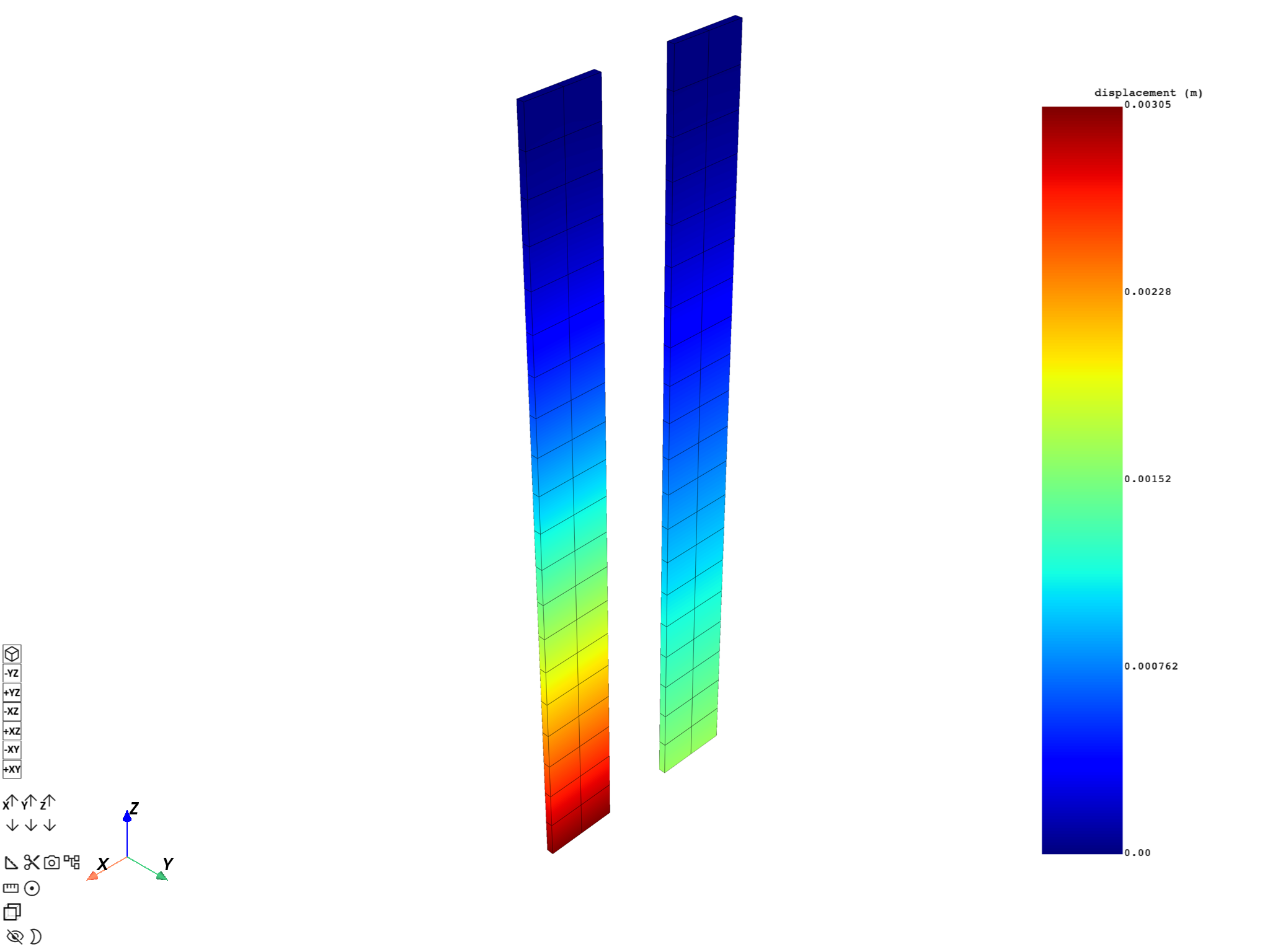# Compare results using the plotter#

This example shows how to plot several mesh/result combinations on the same plot so that you can compare results at different time steps.

```from ansys.dpf import core as dpf
from ansys.dpf.core import examples
from ansys.dpf.core.plotter import DpfPlotter
```

# Compare two results#

Use the `ansys.dpf.core.plotter.DpfPlotter` class to plot two different results over the same mesh and compare them.

```# Here we create a Model and request its mesh
model = dpf.Model(examples.find_msup_transient())

# Then we need to request the displacement for two different time steps
displacement_operator = model.results.displacement()
displacement_operator.inputs.time_scoping.connect([2, 15])
displacement_set2 = displacement_operator.outputs.fields_container()
displacement_set15 = displacement_operator.outputs.fields_container()
```

Use the `ansys.dpf.core.plotter.DpfPlotter` class to add plots for the first mesh and the first result.

```pl = DpfPlotter()

# Create a new mesh and translate it along the x axis.
mesh_set15 = mesh_set2.deep_copy()
overall_field = dpf.fields_factory.create_3d_vector_field(1, dpf.locations.overall)
overall_field.append([0.2, 0.0, 0.0], 1)
coordinates_to_update = mesh_set15.nodes.coordinates_field